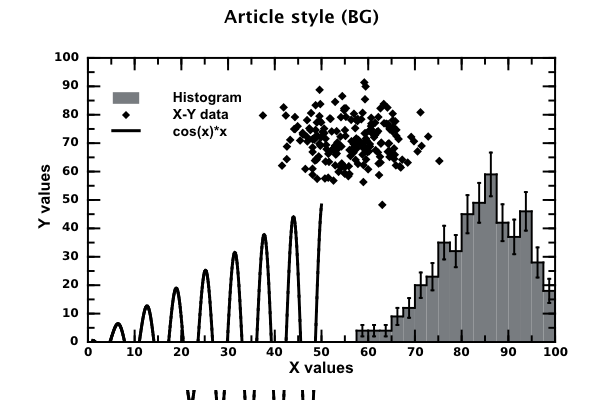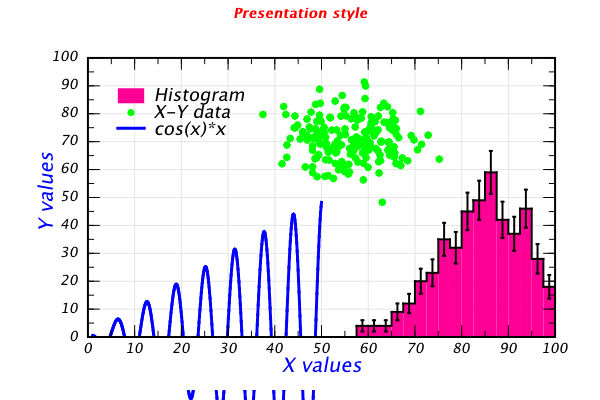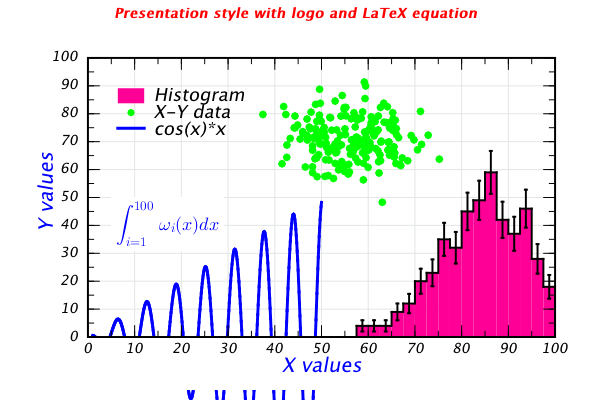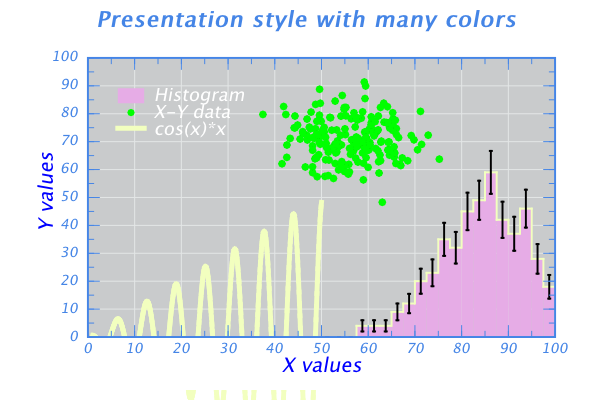# DMelt:Plots/9 Plot Styles by Example

Limitted access. First login to DataMelt if you are a full DataMelt member. Then login to HandWiki as a user.

# Plot styles by example

This tutorial shows various styles of presenting data when using DataMelt. As usual, we make a small Jython code snippets to illustrate various Canvas styles.

The base DataMelt code which makes data for the examples below is the same. It looks as:

from java.util import Random
from jhplot import *

c1 =HPlot("Canvas")
c1.visible()
c1.setRange(0,100,0,100)

h1 = H1D("Histogram",20, 50.0, 100.0)
f1=F1D("cos(x)*x",1,50)
p1= P1D("X-Y data")

rand = Random(10)
for i in range(500):
h1.fill(85+10*rand.nextGaussian())
c1.draw(f1)
c1.draw(h1)
c1.draw(p1)

Below we show how to apply various graphic styles when presenting these 3 objects: a histogram, a function and data points.

## Article styles

Here are "scientific" styles: plots are all in black and while, nothing fancy.The code is shown below:

## Presentation styles

Now we consider alternative styles that use a lot of colors:The code is shown below:

You can put LateX equations too:The code is shown below:

Or you can change background:The code is shown below: